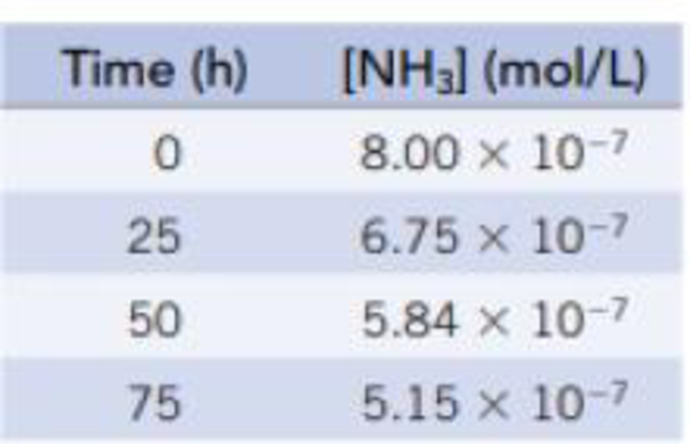Chapter 14, Problem 32PS

Chapter
Section
Textbook Problem

Ammonia decomposes when heated according to the equationNH3(g) → NH2(g) + H(g)The data in the table for this reaction were collected at a high temperature.Plot In [NH3] versus time and 1/[NH3] versus time. What is the order of this reaction with respect to NH3? Find the rate constant for the reaction from the slope.

Interpretation Introduction

Interpretation: ln[NH3] versus time and 1/[NH3] versus time has to be plotted.  The order of this reaction with respect to ammonia.  The rate constant for the reaction from slope has to be found.

Concept Introduction:

The rate of reaction is the quantity of formation of product or the quantity of reactant used per unit time.  The rate of reaction doesn’t depend on the sum of amount of reaction mixture used.

The raise in molar concentration of product of a reaction per unit time or decrease in molarity of reactant per unit time is called rate of reaction and is expressed in units of mol/(L.s).

Integrated rate law for first order reaction:

Consider A as substance, that gives the product based on the equation,

aAproducts

Where a= stoichiometric co-efficient of reactant A.

Consider the reaction has first-order rate law,

Rate=-Δ[A]Δt=k[A]

The integrated rate law equation can be given as,

ln[A]t[A]o=-kt

The above expression is called integrated rate law for first order reaction.

Explanation
• Order of the reaction:

Figure 1

Both graph are linear, the first graph gives the negative slope value whereas second also gives negative value.  The ln[Ammonia] versusTime first order graph which gives negative slope and also linear.  The 1/[Ammonia] versusTime second order graph gives linear but the negative slope (second order graph gives positive slope). Hence, the reaction follows first order.

Calculate the rate constant for the given reaction:

Given:Time(min)ln[NH3](mol/L)08.00×107256.75×107505

Still sussing out bartleby?

Check out a sample textbook solution.

See a sample solution

The Solution to Your Study Problems

Bartleby provides explanations to thousands of textbook problems written by our experts, many with advanced degrees!

Get Started